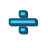Games
Problems
Go Pro!

# Division

Quizzes > Mathematics > Arithmetic > Division

# Featured QuizzesPractice your division facts using this simple quiz. Students who remember their division and multiplication facts complete their work more...Practice your division facts with this simple math quiz from The Problem Site.Practice your division facts with this simple math quiz from The Problem Site.Practice your division facts with this simple quiz at The Problem Site.Practice your division facts with this simple quiz at The Problem Site.Practice your division facts with this simple quiz from The Problem Site.Practice your division with this simple quiz from The Problem Site.Practice your division facts with this simple math quiz from The Problem Site.

# Full Directory Listing

Division by 2, Division by 3, Division by 4, Division by 5, Division by 6, Division by 7, Division by 8, Division by 9, Division Facts (All)Like us on Facebook to get updates about new resources